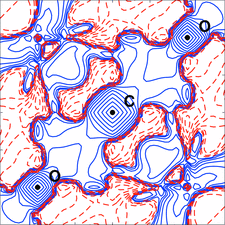# issue contentsFOUNDATIONSADVANCES
ISSN: 2053-2733

# January 2020 issueCover illustration: X-ray diffraction and Compton scattering are equally essential to quantum crystallography because they provide complementary information on the one-electron reduced density matrix (1-RDM) in a crystal. The reconstruction of a 1-RDM solely from X-ray structure factors is bound to yield an inaccurate picture of its off-diagonal regions (as can be seen in the cover image). In this issue, De Bruyne and Gillet [Acta Cryst. (2020), A76, 1-6] describe a method to infer the 1-RDM for a molecular crystal from both experimental data sets while enforcing the necessary N-representability conditions.

## research papers

### Inferring the one-electron reduced density matrix of molecular crystals from experimental data sets through semidefinite programming

In the inference process of finding the one-electron reduced density matrix (1-RDM) of a molecular crystal from diffraction and Compton diffusion scattering data, semidefinite programming can take into account the N-representability conditions on the 1-RDM.

## research papers

### Normally supportive sublattices of crystallographic space groups

Subgroups of 3D crystallographic space groups that are also normal subgroups of 3D space groups have long been of interest. For each arithmetic crystal class of 3D space groups, the sublattices capable of supporting such subgroups are tabulated.

### Cluster-mining: an approach for determining core structures of metallic nanoparticles from atomic pair distribution function data

A novel approach for finding and evaluating structural models of small metallic nanoparticles is presented.

### A combined model of electron density and lattice dynamics refined against elastic diffraction data. Thermodynamic properties of crystalline L-alanine

A combined refinement of a lattice-dynamics and electron-density model is introduced.

### Dynamical effects in the integrated X-ray scattering intensity from imperfect crystals in Bragg diffraction geometry. I. Semi-dynamical model

The sensitivity of the integrated X-ray diffraction intensity to different Coulomb-type defects in real crystals is the subject of theoretical research in the case of Bragg diffraction geometry. The diffraction parameters characterizing defects in the test sample of silicon are determined using the proposed approximate formulas.

### X-ray diffraction from strongly bent crystals and spectroscopy of X-ray free-electron laser pulses

A strongly bent crystal diffracts kinematically when the bending radius is small compared with the critical radius given by the ratio of the extinction length to the Darwin width of the reflection. Under these conditions, the spectral resolution of the X-ray free-electron laser pulse is limited by the crystal thickness and can be better than under dynamical diffraction conditions.

### Monte Carlo simulation of coherently scattered photons based on the inverse-sampling technique

A novel Rayleigh scattering model using anomalous scattering factors based on the inverse-sampling technique was implemented in a general-purpose Monte Carlo radiation transport code. The updated Monte Carlo model provides a more accurate representation of Rayleigh scattering with improved computational efficiency.

### Converting three-space matrices to equivalent six-space matrices for Delone scalars in S6

Given a matrix for transforming vectors in the three-space of unit-cell edge vectors, the corresponding matrix to transform vectors in the six-space of Delone scalars is derived.

### A real-space approach to the analysis of stacking faults in close-packed metals: G(r) modelling and Q-space feedback

A comprehensive direct-space analysis of structural disorder in close-packed metals by simulation of the pair distribution function pattern is described and tested in the case of bulk or nanosized particles.

### Refinement of organic crystal structures with multipolar electron scattering factors

Precomputed multipolar electron scattering factors are employed for electron crystallography. Because this takes into account the fact that atoms are partially charged and aspherical, model fitting statistics and atomic thermal parameters are visibly improved.